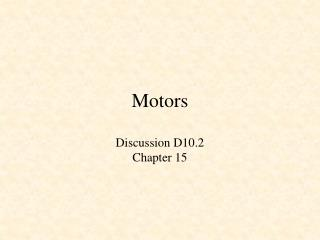DownloadDownload PresentationMotors

# Motors

Download Presentation## Motors

- - - - - - - - - - - - - - - - - - - - - - - - - - - E N D - - - - - - - - - - - - - - - - - - - - - - - - - - -
##### Presentation Transcript

1. Motors Discussion D10.2 Chapter 15

2. Hans Christian Oersted (1777 – 1851) X 1822 In 1820 he showed that a current produces a magnetic field. Ref: http://chem.ch.huji.ac.il/~eugeniik/history/oersted.htm

3. André-Marie Ampère (1775 – 1836) French mathematics professor who only a week after learning of Oersted’s discoveries in Sept. 1820 demonstrated that parallel wires carrying currents attract and repel each other. attract A moving charge of 1 coulomb per second is a current of 1 ampere (amp). repel

4. What do Maxwell’s Eqs. Predict? B = magnetic flux density (magnetic induction) m = magnetic permeability Magnetic field lines must be closed loops Force on moving charge q Lorentz force B

5. Vector Multiplication Scalar (Dot) Product Vector (Cross) Product A A q q B B Direction of C given by "Right-hand rule" (into the screen)

6. Force on current in a magnetic field Force on moving charge q -- Lorentz force Current density, j, is the amount of charge passing per unit area per unit time. N = number of charges, q, per unit volume moving with mean velocity, v. j Force per unit length on a wire is

7. B Force out i + - i Force in X Rotating Machine

8. X Rotating Machine B Force in i - + i Force out

9. B Force out commutator i + - i brushes Force in X Rotating Machine

10. What do Maxwell’s Eqs. Predict? Corresponds to Faraday’s law of electromagnetic induction A changing magnetic flux B density induces a curl of E

11. Back emf X X B B Force out r i a q l + - b i Force in

12. B Force out i + - i Force in X Back emf a b

13. B Force out commutator i + - i brushes Force in X Back emf

14. X X X X Armature with four coil loops S N

15. Motor Circuit Power and Torque

16. Armature Current, Speed, Power and Torque

17. FF-130 Series Motors

18. Maximum Power

19. Generator Circuit

20. Motor Driver Circuit

21. MOS FET Relays G3VM-61B1

22. Pulse-Width Modulation

23. pwm.v module pwm(clk,clr,duty,pwm); input clk; input clr; input [3:0] duty; output pwm; reg [3:0] count; reg pwm; wire set, reset; // 4-bit counter always @(posedge clk or posedge clr) if(clr == 1) count <= 0; else count <= count + 1;

24. pwm.v assign set = ~| count; assign reset = (count == duty); always @(posedge clk) begin if(set == 1) pwm <= 1; if(reset == 1) pwm <= 0; end endmodule

25. Lab 11

26. Lab 11